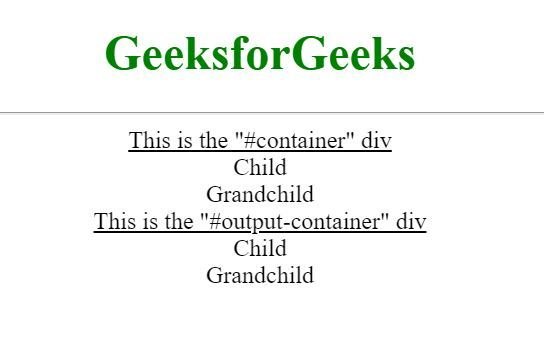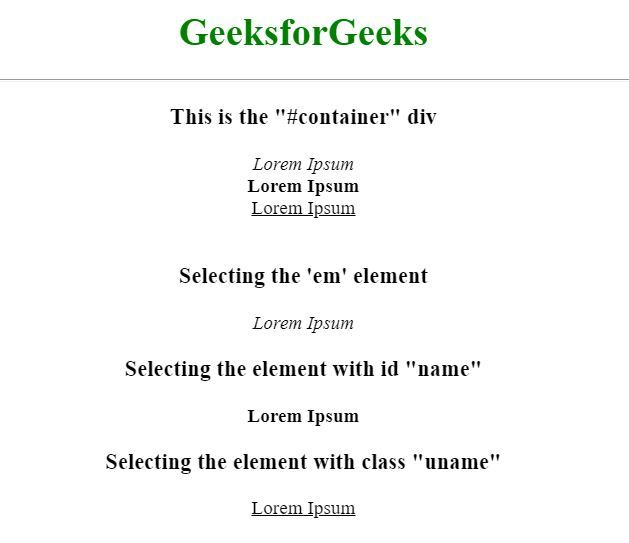Open in App
Not now

# How to iterate through child elements of a div using jQuery ?

• Last Updated : 03 Aug, 2021

jQuery Selector can be used to find (select) HTML elements from the DOM. Once an element is selected, the jQuery children() method is called to find all the child elements of the selected element. To loop through the child elements, the jQuery each() method is used as demonstrated in the following example.

Example 1: The sample HTML page contains a “container” div having some child elements. Looping is done throughout all the child elements of the “container” div and then copied them to the “output-container” div which initially had no child elements.

 ```<``html``>`` ` `<``head``>``    ````    ``<``script` `src``=``"https://code.jquery.com/jquery-3.5.1.min.js"``>``    `````` ` `<``body` `style``=``"text-align: center;"``>``    ``<``h1` `style``=``"color: #008000"``>``        ``GeeksforGeeks``    ````    ``<``hr``>`` ` `    ``<``u``>This is the "#container" div`` ` `    ``<``div` `id``=``"container"``>``        ``<``div``>``            ``Child``            ``<``div``>``                ``Grandchild``            ````        ````    ```` ` `    ``<``u``>This is the "#output-container" div``    ``<``div` `id``=``"output-container"``>`` ` `    ``<``script``>``        ``// loop through the child elements``        ``// of the "#container" div``        ``\$("#container").children()``                ``.each(function () {`` ` `            ``// "this" is the current child``            ``// in the loop grabbing this ``            ``// element in the form of string``            ``// and appending it to the ``            ``// "#output-container" div``            ``var element = \$(this)``                    ``.prop('outerHTML');`` ` `            ``\$("#output-container")``                    ``.append(element);``        ``});``    `````` ` ``

Output:Example 2: In the above example, looping is implemented through all the child elements. But if it is required to loop for some specific child elements or elements with some specific class or id, then it is passed as an argument to the children() function as demonstrated below.

 ```<``html``>`` ` `<``head``>``    ````    ``<``script` `src``=``"https://code.jquery.com/jquery-3.5.1.min.js"``>``    `````` ` `<``body``>``    ``<``h1` `style``=``"color: #008000"``>``        ``GeeksforGeeks``    ````    ``<``hr``>`` ` `    ``<``h3``>This is the "#container" div`` ` `    ``<``div` `id``=``"container"``>``        ``<``em``>Lorem Ipsum<``br``>``        ``<``strong` `id``=``"name"``>``            ``Lorem Ipsum``        ``<``br``>`` ` `        ``<``u` `class``=``"uname"``>Lorem Ipsum``    ````    ``<``br``>`` ` `    ``<``h3``>Selecting the 'em' element``    ``<``div` `id``=``"em-container"``>`` ` `    ``<``h3``>``        ``Selecting the element ``        ``with id "name"``    ````    ``<``div` `id``=``"name-container"``>`` ` `    ``<``h3``>``        ``Selecting the element ``        ``with class "uname"``    ````    ``<``div` `id``=``"uname-container"``>`` ` `    ``<``script``>`` ` `        ``// looping over the child 'em' ``        ``// elements of the "#container" div``        ``\$("#container").children('em').each(function()``        ``{``            ``var element = \$(this).prop('outerHTML');``            ``\$("#em-container").append(element);``        ``});`` ` `        ``// looping over the child elements of``        ``// the "#container" div having id as "name"``        ``\$("#container").children('#name').each(function()``        ``{``            ``var element = \$(this).prop('outerHTML');``            ``\$("#name-container").append(element);``        ``});`` ` `        ``// looping over the child elements of``        ``// the "#container" div having class as "uname"``        ``\$("#container").children('.uname').each(function()``        ``{``            ``var element = \$(this).prop('outerHTML');``            ``\$("#uname-container").append(element);``        ``});``    `````` ` ``

Output:The above example demonstrates all the three cases, looping over specific child elements, looping over child elements with a specific id, and looping over child elements with a specific class.

jQuery is an open source JavaScript library that simplifies the interactions between an HTML/CSS document, It is widely famous with it’s philosophy of “Write less, do more”.
You can learn jQuery from the ground up by following this jQuery Tutorial and jQuery Examples.

My Personal Notes arrow_drop_up